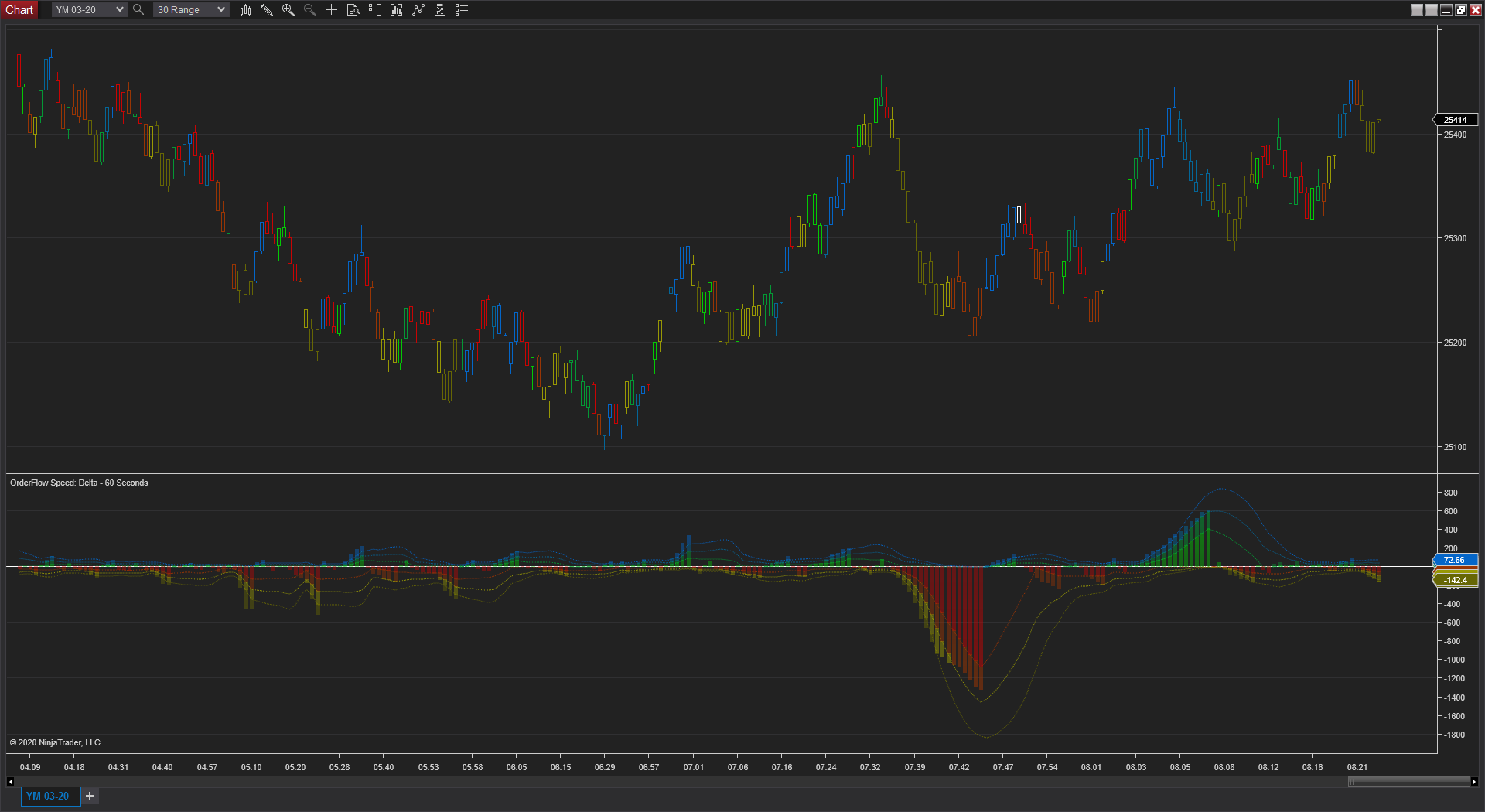To properly display this page you need a browser with JavaScript support.
×
 OrderFlow Speed OrderFlow Speed - Parameters

# OrderFlow Speed - Parameters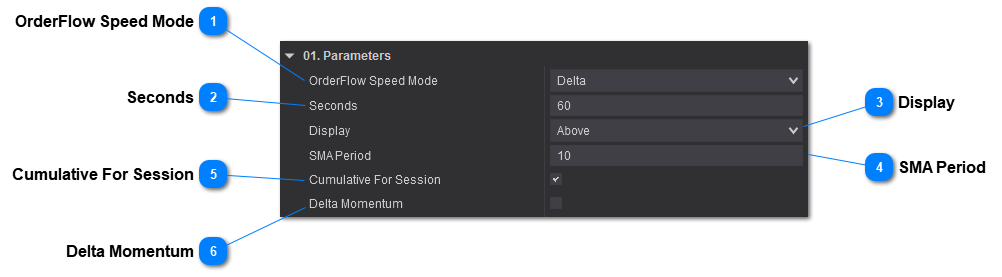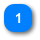## OrderFlow Speed ModeSets the type of orderflow volume.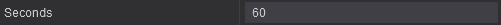Sets the number of seconds of the moving window to calculate the volume.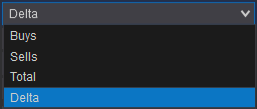Will calculate the speed at which the buys take place.

## Sells -

Will calculate the speed at which the sells take place.

## Total -

Will calculate the speed at which the total volume (buys and sells) take place.

## Delta -

Will calculate the speed at which the delta volume (buys minus sells) take place. This will override the Display setting and will display both above (positive delta) and below (negative delta).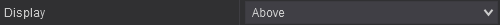Sets where the values are be plotted for Modes - Buys, Sells, Total, in reference to Zero.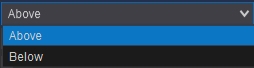# Chart Displaying diferent Modes and Display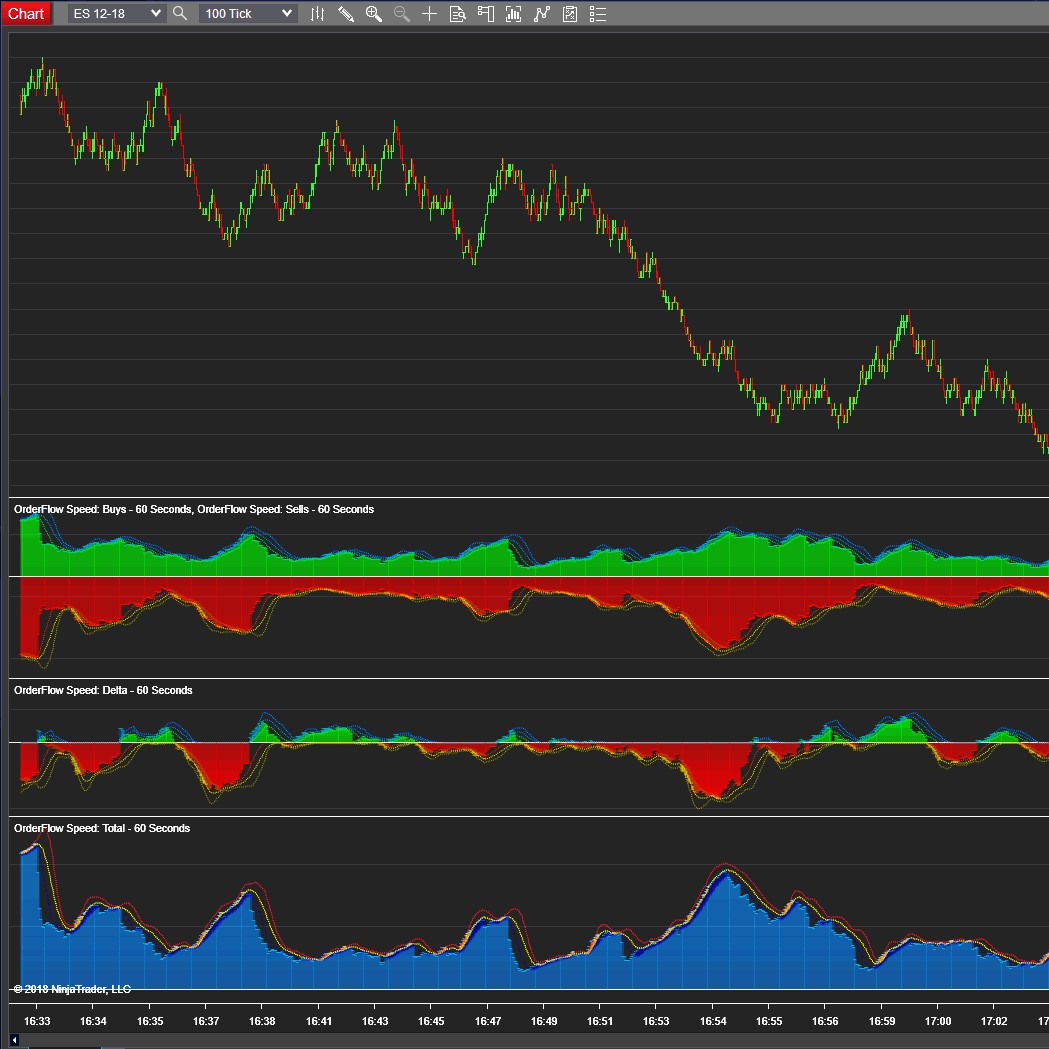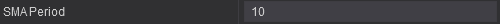Sets the period for the SMA and its Standard Deviations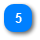## Cumulative For Session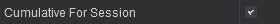Will reset the OrderFlow Speed at Session Start. Will show a cumulative OrderFlow Speed for the session.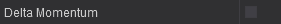Will provide a momentum type of chart based on the orderflow speed. Will keep adding the number of contracts until the direction of OrderFlow Speed changes direction. See a example below.
Will not display Momentum if you have Cumulative For Session set to true as Cumuolative Session will override by acumulating per session and not per direction.
Will work when OrderFlow Speed Mode is set to Delta, otherwise will be ignored.